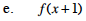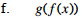### Home > PC > Chapter 1 > Lesson 1.1.3 > Problem1-41

1-41.
1. Given f(x) = x2 + 2x and g(x) =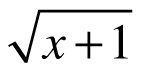, find the following: Homework Help ✎

1. g(x − 2)

2. f(g(8))

3. g(f(8))

4. f(f(1))

5. f(x + 1)

6. g(f(x))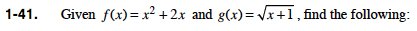Replace every x in the g function with the expression (x − 2). Then simplify.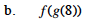Find g(8) first. Then replace every x in the f function with the value of g(8). Simplify.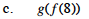Find f(8) first. Then replace every x in the g function with the value of f(8). Simplify.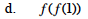Find f(1) first. Then replace every x in the f function with the value of f(1). Simplify.

15